## Ratio and Proportion For SBI PO : Set – 08

1) One year ago the ratio between rahul salary and rohit salary is 4:5. The ratio between their individual salary of the last year and current year is 2:3 and 3:5 respectively. If the total current salary of rahul and rohit is 4300. Then find the current salary of rahul.

a) 1200

b) 1800

c) 2400

d) 3600

e) None of these

b)

4x and 5x is the last year salry of rahul and rohit respectively
Rahul last year to rahul current year = 2/3
Rohit last year to rohit current year = 3/5
Current of rahul + current of rohit = 4300
(3/2)*4x + (5/3)*5x = 4300.
X = 300.
So rahul current salary = 3/2 * 4* 300 = 1800

2) The ratio between the present age of Maha and Deepa is 5:X. Maha is 9yrs younger than Parveen.Parveen’s age after 9yrs will be 33yrs. The difference between the Deepa and Maha age is same as the present age of Parveen.Find X.

a) 13

b) 10

c) 11

d) 14

e) None of these

a)

P present age = 33-9 = 24
M = 24 – 9 =15
D – M = 24
D – 15 = 24
D = 24+15 = 39
15:39 = 5:13
X = 13

3) A bag contains 25p coins, 50p coins and 1 rupee coins whose values are in the ratio of 8:4:2. The total values of coins are 840. Then find the total amount in rupees.

a) 220

b) 240

c) 260

d) 280

e) None of these

d)
Explanation :
Value is given in the ratio 8:4:2.
(8x/0.25) + (4x/0.5) + (2x/1) = 840.
X = 20. Total amount = 14*20 = 280

4) The sum of the squares of three numbers is 532and the ratio of the first and the second as also of the second and the third is 3:2.then the first number is

a) 8

b) 12

c) 18

d) 20

e) none of these

c)

From the question a : b = 3 : 2 & b : c = 3 : 2

So a : b : c = 9 : 6 : 4

And also (9x)2 + (6x)2 + (4x)2 = 532

133 x2 = 532

X= 4

X = 2

Therefore first number is 18.

5) If ab =36 ,which of the following is proportions is correct?

a) 9:a=4:b

b) a:18 =b:3

c) a:6=b:6

d) a:9=4:b

e) none of these

d)

a:9 = 4:b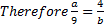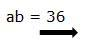6) Two numbers are in the ratio of 1: 2. If 7 is added to both the number their ratio changes to 3:5. The greater number is

a) 24

b) 36

c) 28

d) 32

e) None of these

c)

Let the two numbers be x, 2x.

When 7 is added to both the numbers, we get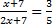5x + 35 = 6x + 21

X = 14

The greatest number is, 2x = 2 *14

=28

7) A, B and C jointly started a business. A got 3/5 part of the whole profit. The remaining profit was distributed equally between B and C. If C got Rs. 400 less than A then find the total amount of Profit.

a) Rs. 1600

b) Rs. 1200

c) Rs. 1000

d) Rs. 800

e) None of these

c)

Let the total profit be Rs. x

Then A’s Share sum profit = Rs.3/5x

Remaining Profit = x-3x/5

2x/5

Therefore B’s Share Sum profit =Rs.x/5

C’s share Sum Profit = Rs.x/5

According to the question,

(3x/5-x/5)=400

2x/5=400

x=(400*5)/2

x= 1000

8) The ratio of the numbers of boys and girls in a school was 5 : 3. Some new boys and girls were admitted to the school, in the ratio 5 : 7. At this, the total number students in the school became 1200, and the ratio of boys to girls changed to 7:5. The number of students in the school before new admissions was

a) 700

b) 720

c) 900

d) 960

e) None of these

d)

(5X+5Y)/(3X+7Y)=7/5

2X-12Y=0 ——->1

Initially, 5X+3X+5Y+7Y=1200

2X+3Y=300 ———->2

Solving 1 and 2, X=120; Y=20

Required Students=8X=8 x 120=960

9) The ratio of the fares of the first and second class between two railway stations is 4 : 1 and that of the number of passengers travelling by first and second classes is 1 : 40. If on a day Rs. 1,100 are collected as total fare, the amount collected from the first class passengers is

a) Rs. 315

b) Rs. 275

c) Rs. 137.50

d) Rs. 100

e) None of these

d)

Fare of first class=4X

Fare of second class=X

Number of travellers in first class=Y

Number of travellers in second class=40Y

Total Fare=1100

4XY+40XY=1100

XY=25

Required Amount=4XY=4×25=100

10) In what ratio must a person mix three kinds of Oats costing him Rs 1.20,Rs 1.44 and Rs 1.74 per Kg so that the mixture may be worth Rs 1.41 per Kg?

a)11:77:7

b) 11:45:7

c) 25:45:8

d) 27:23:6

e) None

1.20   1.44   1.74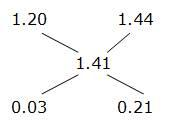3:21 -> 1:7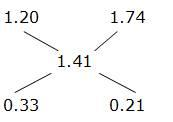33:21 -> 11:7

1:7

11:7

⇒ 11:77:7